Function Repository Resource:

# KoremutakeConvert

Convert to and from Koremutake memorable random strings

Contributed by: Anton Antonov
 ResourceFunction["KoremutakeConvert"][n] converts the integer n into a Koremutake memorable string. ResourceFunction["KoremutakeConvert"][s] converts the given Koremutake string s into an integer.

## Details

Koremutake representation is a way to express any large number as a sequence of syllables. The general idea is that pieces of information that sound like words are a lot easier to remember than a sequence of digits.
ResourceFunction["KoremutakeConvert"] can take integers and strings as arguments.
String case is ignored.
Representations of negative integers are prefixed with "minus".
The syllable-to-integer mapping can be obtained by using "SyllableMapping" as an argument.
The integer-to-syllable mapping can be obtained by using "IntegerMapping" as an argument.

## Examples

### Basic Examples (3)

Convert an integer into a Koremutake memorable string:

 In:=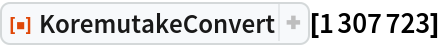Out=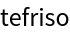Convert a negative integer:

 In:=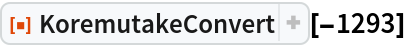Out=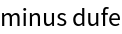Convert a Koremutake string into an integer:

 In:=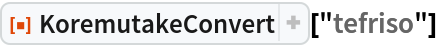Out=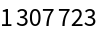### Scope (3)

The function Koremutake works on lists (it has the attribute Listable):

 In:=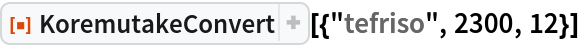Out=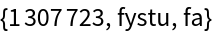Get the syllable-to-integer mapping:

 In:=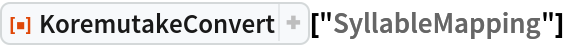Out=Get the integer-to-syllable mapping:

 In:=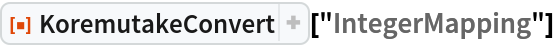Out=### Applications (2)

Generate memorable strings for a list of large primes:

 In:=Out=Convert the obtained memorable strings into integers:

 In:=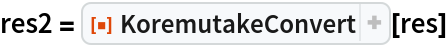Out=### Properties and Relations (4)

Random integers can be converted to memorable strings:

 In:=Out=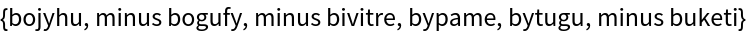Using the resource function Soundex, you can "generalize" the Koremutake representations into Soundex codes:

 In:=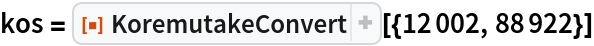Out=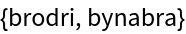In:=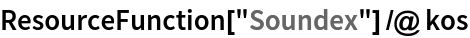Out=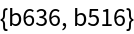Note that variations of "dribro" and "branaby" are represented with the same Soundex codes:

 In:=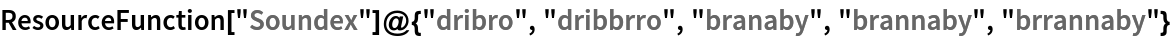Out=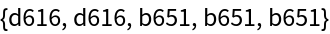The variations with "too many" consonants are not recognized by KoremutakeConvert:

 In:=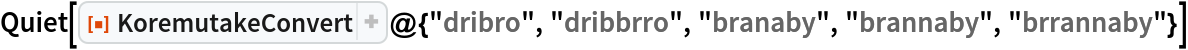Out=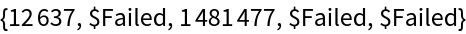Here a random tabular dataset is generated with the resource function RandomTabularDataset, which has a "Koremutake" column:

 In:=Out=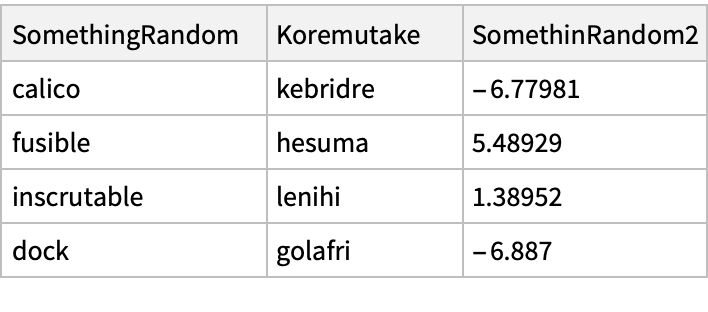KoremutakeConvert is self-inverting:

 In:=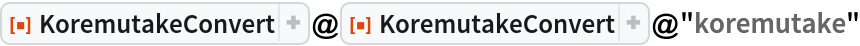Out=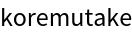### Neat Examples (3)

Get the number represented by "Koremutake", find the list of prime factors and represent those factors with Koremutake strings:

 In:=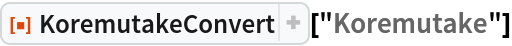Out=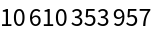In:=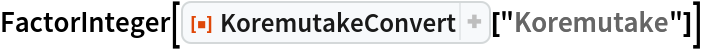Out=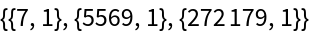In:=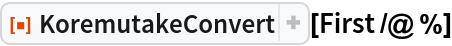Out=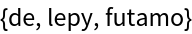Find pet names that are valid Koremutake strings:

 In:=Out=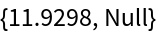Here is the number of pet names that are Koremutake strings per species:

 In:=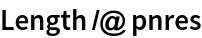Out=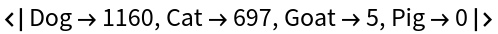Here are samples per species of Koremutake pet names:

 In:=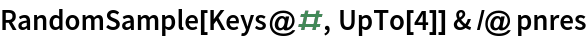Out=Generate random numbers:

 In:=Out=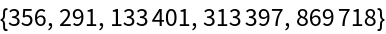Convert to Koremutake strings and make a "phrase":

 In:=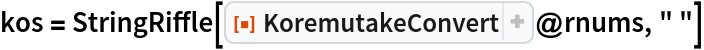Out=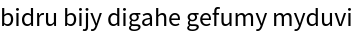Speak the Koremutake representations of the random numbers:

 In:=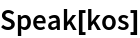Anton Antonov

## Version History

• 1.0.0 – 16 November 2021

## Author Notes

See shorl.com for more detailed explanations.